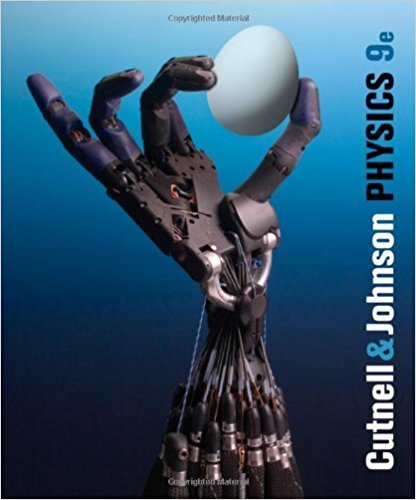×

×

# Solutions for Chapter 10: Simple Harmonic Motion and Elasticity## Full solutions for Physics, | 9th Edition

ISBN: 9780470879528Solutions for Chapter 10: Simple Harmonic Motion and Elasticity

Solutions for Chapter 10
4 5 0 260 Reviews
19
5
##### ISBN: 9780470879528

Chapter 10: Simple Harmonic Motion and Elasticity includes 146 full step-by-step solutions. This expansive textbook survival guide covers the following chapters and their solutions. This textbook survival guide was created for the textbook: Physics,, edition: 9. Physics, was written by and is associated to the ISBN: 9780470879528. Since 146 problems in chapter 10: Simple Harmonic Motion and Elasticity have been answered, more than 200069 students have viewed full step-by-step solutions from this chapter.

Key Physics Terms and definitions covered in this textbook
• //

parallel

• any symbol

average (indicated by a bar over a symbol—e.g., v¯ is average velocity)

• °C

Celsius degree

• °F

Fahrenheit degree